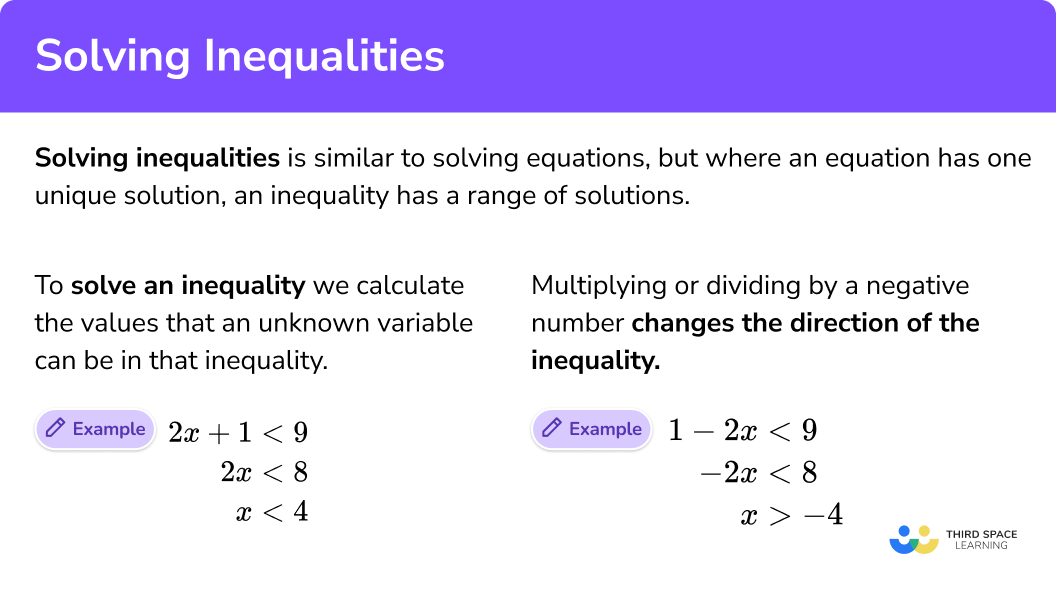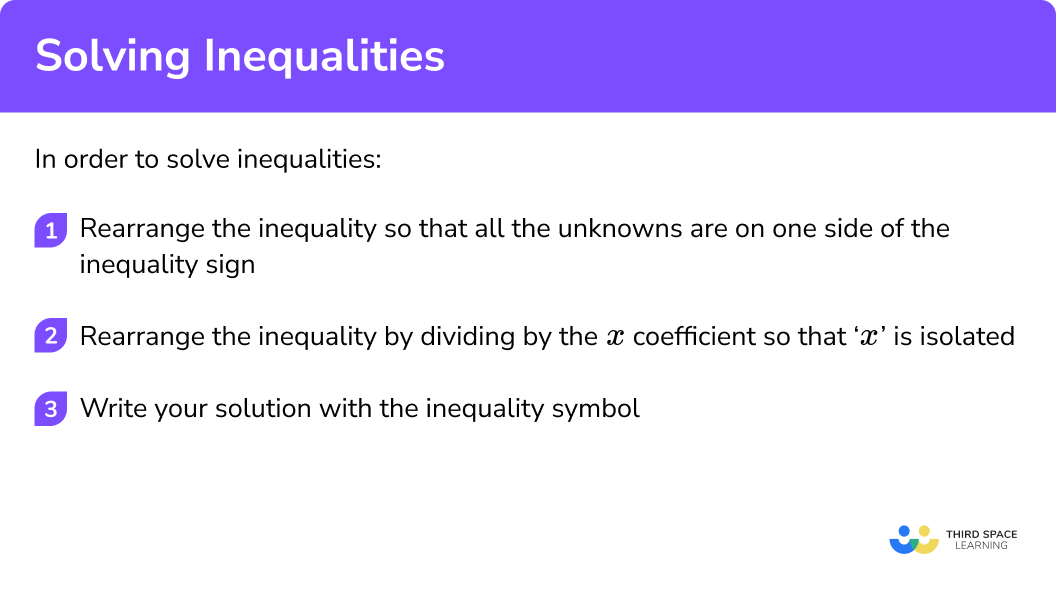GCSE Maths Algebra Inequalities

Solving Inequalities

# Solving Inequalities

Here we will learn about solving inequalities including how to solve linear inequalities, identify integers in the solution set and represent solutions on a number line.

There are also solving inequalities worksheets based on Edexcel, AQA and OCR exam questions, along with further guidance on where to go next if you’re still stuck.

## What is solving inequalities?

Solving inequalities is where we calculate the values that an unknown variable can be in an inequality.

Solving inequalities is similar to solving equations, but where an equation has one unique solution, an inequality has a range of solutions.

To do this we need to balance the inequality in the same way as we would when solving an equation. Solutions can be integers or decimals, positive or negative numbers.

### What is solving inequalities?### Solving an equation

Solve

\begin{aligned} 2x+1&=9\\ 2x&=8\\ x&=4 \end{aligned}

4 is the only solution to this equation.

2 × 4 + 1 = 9

### Solving an inequality

Solve

\begin{aligned} 2x+1&<9\\ 2x&<8\\ x&<4 \end{aligned}

x can be any value that is less than 4

### Multiplying and dividing by a negative number

This changes the direction of the inequality sign.

E.g.

\begin{aligned} 1 – 2x&< 9\\ -2x&< 8\\ x&>-4 \end{aligned}

x can be any value that is greater than -4

## How to solve inequalities

In order to solve inequalities:

1. Rearrange the inequality so that all the unknowns are on one side of the inequality sign.
2. Rearrange the inequality by dividing by the x coefficient so that ‘x’ is isolated.
3. Write your solution with the inequality symbol.

### Explain how to solve inequalities## Related lessons on inequalities

Solving inequalities is part of our series of lessons to support revision on inequalities. You may find it helpful to start with the main inequalities lesson for a summary of what to expect, or use the step by step guides below for further detail on individual topics. Other lessons in this series include:

## Solving linear inequalities examples

### Example 1: solving linear inequalities

Solve

4x+6 < 26

1. Rearrange the inequality so that all the unknowns are on one side of the inequality sign.

In this case you are subtracting ‘6’ from both sides.

\begin{aligned} 4x+6&<26\\ 4x&<20\\ \end{aligned}

2Rearrange the inequality by dividing by the x coefficient so that ‘x’ is isolated.

In this case you need to divide both sides by 4 .

\begin{aligned} 4x+6&<26\\ 4x&<20\\ x&<5 \end{aligned}

3Write your solution with the inequality symbol.

x < 5

Any value less than 5 satisfies the inequality

### Example 2: solving linear inequalities

Solve

5x-4\geq26

Rearrange the inequality so that all the unknowns are on one side of the inequality sign.

Rearrange the inequality by dividing by the x coefficient so that ‘x’ is isolated.

Write your solution with the inequality symbol.

### Example 3: solving linear inequalities with brackets

Solve

3(x-4)\leq12

Rearrange the inequality so that all the unknowns are on one side of the inequality sign.

Rearrange the inequality by dividing by the x coefficient so that ‘x’ is isolated.

Write your solution with the inequality symbol.

### Example 4: solving linear inequalities with unknowns on both sides

Solve

5x - 6 > 2x + 15

Rearrange the inequality so that all the unknowns are on one side of the inequality sign.

Rearrange the inequality so that ‘x’s are on one side of the inequality sign and numbers on the other.

Rearrange the inequality by dividing by the x coefficient so that ‘x’ is isolated.

Write your solution with the inequality symbol.

### Example 5: solving linear inequalities with fractions

Solve

\frac{x+3}{5}<2

Rearrange the inequality to eliminate the denominator.

Rearrange the inequality so that ‘x’s are on one side of the inequality sign and numbers on the other.

Write your solution with the inequality symbol.

### Example 6: solving linear inequalities – non integer solutions

Solve

6x+1\geq4

Rearrange the inequality so that all the unknowns are on one side of the inequality sign.

Rearrange the inequality by dividing by the x coefficient so that ‘x’ is isolated.

Write your solution with the inequality symbol.

### Example 7: solving linear inequalities and representing solutions on a number line

Represent the solution on a number line

2x - 7 < 5

Rearrange the inequality so that ‘x’s are on one side of the inequality sign and numbers on the other.

Rearrange the inequality by dividing by the x coefficient so that ‘x’ is isolated.

Represent your solution on a number line.

### Example 8: solving linear inequalities with negative x coefficients

Solve

1 - 2x <7

Rearrange the inequality so that ‘x’s are on one side of the inequality sign and numbers on the other.

Rearrange the inequality by dividing by the x coefficient so that ‘x’ is isolated.

Change the direction of the inequality sign.

### Example 9: solving linear inequalities and listing integer values that satisfy the inequality

List the integer values that satisfy

3<x+1\leq8

Rearrange the inequality so that all the unknowns are on one side of the inequality sign.

List the integer values satisfied by the inequality.

### Example 10: solving linear inequalities and listing integer values that satisfy the inequality

List the integer values that satisfy

7\leq4x\leq20

Rearrange the inequality so that all the unknowns are on one side of the inequality sign.

List the integer values satisfied by the inequality.

### Example 11: solving linear inequalities and representing the solution on a number line

List the integer values that satisfy

-3<2x+5\leq7

Rearrange the inequality so that all the unknowns are on one side of the inequality sign.

Rearrange the inequality so that ‘x’ is isolated.  In this case you need to divide each part by 2.

Represent the solution set on a the number line

### Common misconceptions

• Solutions as inequalities

Not including the inequality symbol in the solution is a common mistake. An inequality has a range of values that satisfy it rather than a unique solution so the inequality symbol is essential

E.g.

When solving x + 3 < 7 giving a solution of ‘4’ or ‘x = 4’ is incorrect, the answer must be written as an inequality ‘x < 4’

• Balancing inequalities

Errors can be made with solving equations and inequalities by not applying inverse operations or not balancing the inequalities. Working should be shown step-by-step with the inverse operations applied to both sides of the inequality.

E.g.

When solving x + 3 < 7 , adding ‘3’ to both sides rather than subtracting ‘3’ from both sides

### Practice solving inequalities questions

1. Solve 3x+7 < 31

3x < 24x > 88x < 8\begin{aligned} 3x+7&<31\\ 3x&<24\\ x&<8 \end{aligned}

2. Solve 4x-3\geq25

x < 7x\geq7x > 77\begin{aligned} 4x-3&\geq25\\ 4x&\geq28\\ x&\geq7\\ \end{aligned}

3. Solve 2(x-5)\leq8

x < 9x\leq-1x\leq99\begin{aligned} 2(x-5)&\leq8\\ 2x-10&\leq8\\ 2x&\leq18\\ x&\leq9\\ \end{aligned}

4. Solve 6x – 5 > 4x + 1

x > 3x < 3.5x\leq33\begin{aligned} 6x-5&>4x+1\\ 2x-5&>1\\ 2x&>6\\ x&>3\\ \end{aligned}

5. Solve \frac{x-4}{2}>6

x < 4x >16x\leq316\begin{aligned} \frac{x-4}{2}>6\\ x-4&>12\\ x&>16\\ \end{aligned}

6. Solve 8x+1\geq3

x\geq4x\leq4\frac{1}{4}x\geq\frac{1}{4}\begin{aligned} 8x+1&\geq3\\ 8x&\geq2\\ x&\geq\frac{2}{8}\\ x&\geq\frac{1}{4}\\ \end{aligned}

7. Represent the solution on a number line 5x – 2 < 28\begin{aligned} 5x-2&<28\\ 5x&<30\\ x&<6\\ \end{aligned}

An open circle is required and all values less than 6 indicated.

8. Solve 2 – 3x > 14

x > 4x < 4-x > 4x<\frac{16}{3}\begin{aligned} 2- 3x&>14\\ -3x&>12 \\ x&< 4\\ \end{aligned}

Change the direction of the inequality sign as you have divided by a negative number

9. List the integer values that satisfy 2<x+3\leq5

0, 1, 22, 3, 4, 53, 4, 5-1, 0, 1, 2\begin{aligned} 2<x+3\leq5\\ -1<x\leq2\\ \end{aligned}

-1 is not included in the solution set as is greater than -1 .
2 is included in the solution set as x is less than or equal to 2 .

10. List the integer values that satisfy 4\leq3x\leq21

1, 2, 3, 4, 5, 6, 72, 3, 4, 5, 61, 2, 3, 4, 5, 62, 3, 4, 5, 6, 7\begin{aligned} 4\leq3 x \leq21\\ \frac{4}{3}\leq x \leq7\\ \end{aligned}

The first integer greater than \frac{4}{3} is 2 .
7 is included in the solution set as x is less than or equal to 7 .

11. List the integer values that satisfy -4<3x+2\leq5

-2, -1, 0, 1-1, 0-1, 0, 1-3, -2, -1, 0, 1, 2, 3, 4, 5\begin{aligned} -4<3x+2\leq5\\ -6<3x\leq3\\ -2<x\leq1\\ \end{aligned}

-2 is not included in the solution set as x is greater than -2 .
1 is included in the solution set as x is less than or equal to 1 .

### Solving inequalities GCSE questions

1. John’s solution to 2x + 5 > 17 is shown on the number lineIs John’s solution correct?

(2 marks)

Correctly solves the inequality

(1)

No, correct solution is x > 6

Indicates ‘no’ with correct reason or represents correct inequality on the number line

(1)

2. (a) Solve 4x+1\leq3x-2
(b) Represent your solution to (a) on the number line

(4 marks)

(a)
Correct attempt at solving, for example eliminating ‘x’ . x+1\leq-2

(1)

Correct solution x\leq-3

(1)

(b)
‘-3’ or their value indicated on the number line with a closed circle

(1)

Correct inequality or their inequality shown on the number line with aa closed circle and values on the left side of the circle indicated with an arrow.

(1)

3. (a) Solve 5x – 1 > 9
(b) Write down the smallest integer that satisfies 5x – 1 > 9

(3 marks)

(a)
Correct attempt at solving: 5x > 10

(1)

Correct solution: x > 2

(1)

(b)
Correct solution: 3

(1)

## Learning checklist

You have now learned how to:

• Solve inequalities
• Represent your solutions on a number line
• List integer values that satisfy your inequality

## Still stuck?

Prepare your KS4 students for maths GCSEs success with Third Space Learning. Weekly online one to one GCSE maths revision lessons delivered by expert maths tutors.

Find out more about our GCSE maths tuition programme.